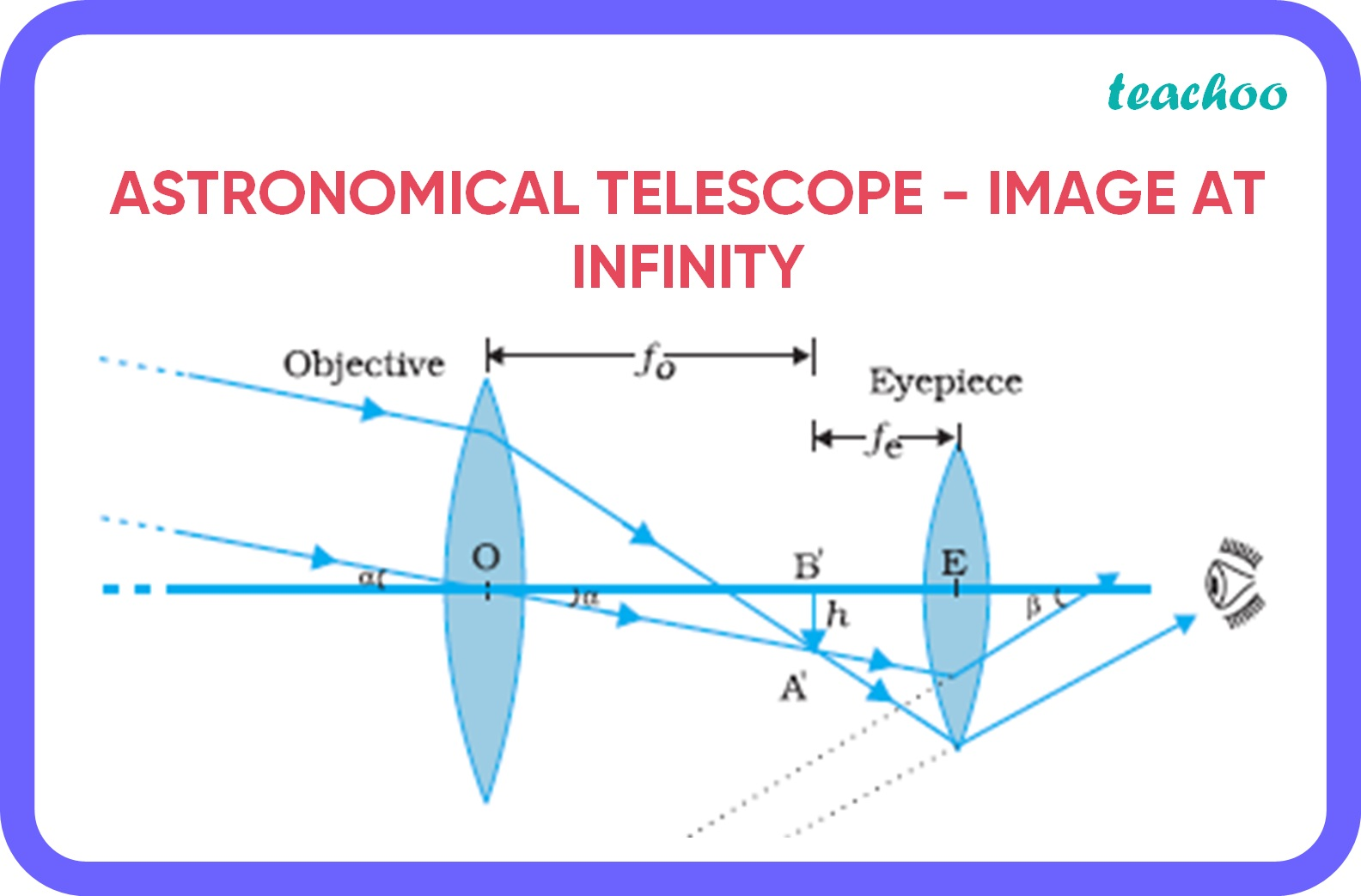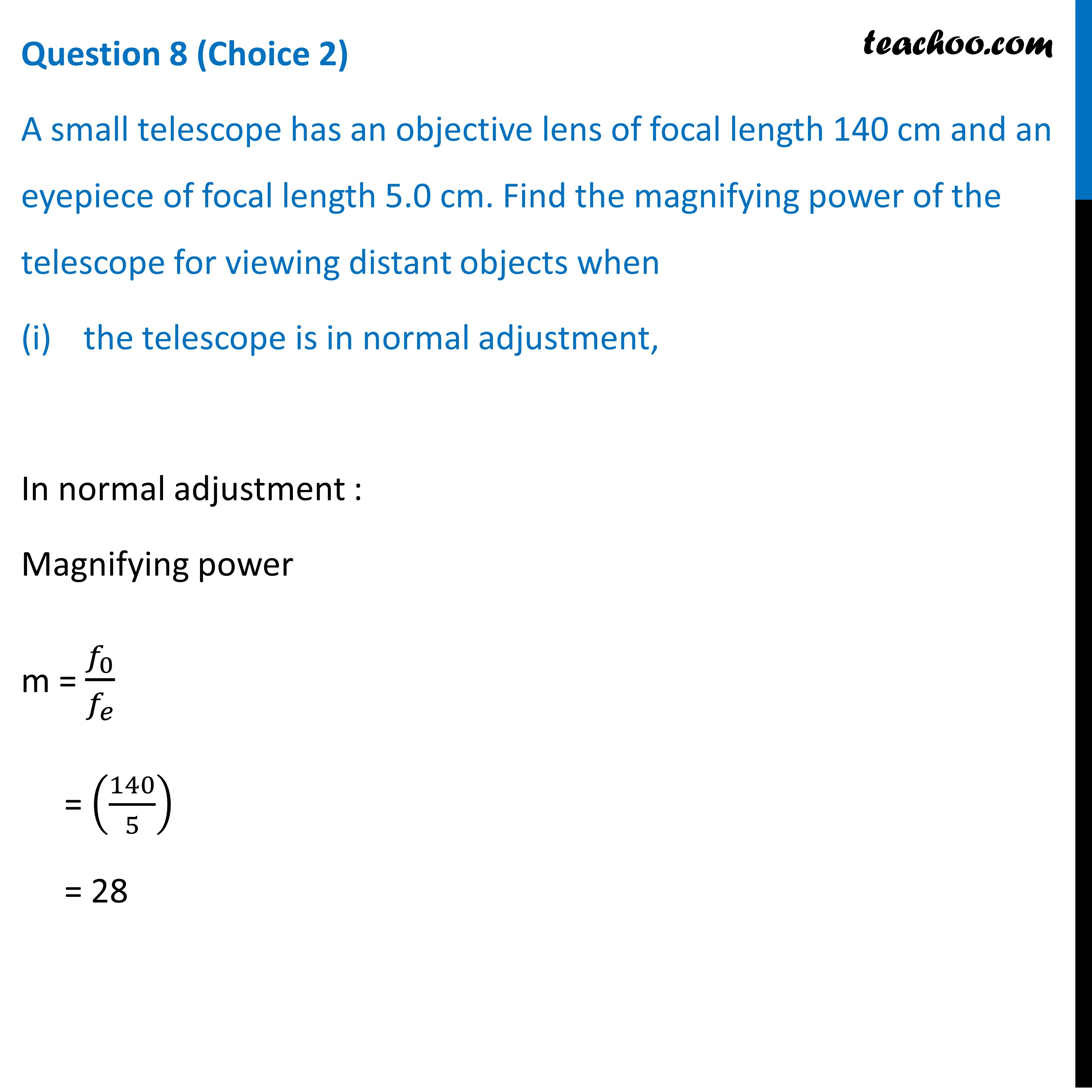CBSE Class 12 Sample Paper for 2022 Boards [Term 2] - Physics

Physics Class 12
Solutions to CBSE Sample Paper - Physics Class 12

## (a) Draw a ray diagram of the Astronomical Telescope for the final image formed at infinity.

Ray diagram of astronomical telescope when image is formed at infinity.## (i) the telescope is in normal adjustment,## (ii) the final image is formed at the least distance of distinct vision.Learn in your speed, with individual attention - Teachoo Maths 1-on-1 Class

### Transcript

Question 8 (Choice 2) A small telescope has an objective lens of focal length 140 cm and an eyepiece of focal length 5.0 cm. Find the magnifying power of the telescope for viewing distant objects when the telescope is in normal adjustment, In normal adjustment : Magnifying power m = 𝑓_0/𝑓_𝑒 = (140/5) = 28 Question 8 (Choice 2) A small telescope has an objective lens of focal length 140 cm and an eyepiece of focal length 5.0 cm. Find the magnifying power of the telescope for viewing distant objects when (ii) the final image is formed at the least distance of distinct vision. When the final image is formed at the least distance of distinct vision (25 cm) m = 𝑓_0/𝑓_𝑒 (1+𝑓_𝑒/𝐷) = (28×1.2) = 33.6